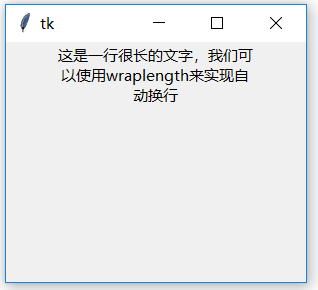4.1 标签控件的创建
4.1.1 单行文本

``````import tkinter as tk
root=tk.Tk()
root.geometry('300x240')
b1 = tk.Label(root,text='我是一个标签')
b1.pack()
root.mainloop()
``````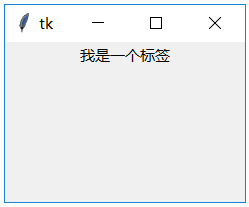4.1.2 显示图像

``````import tkinter as tk

root=tk.Tk()
root.geometry('300x240')

p = tk.PhotoImage(file='a.gif')
b1 = tk.Label(root,image=p)
b1.pack()

root.mainloop()
``````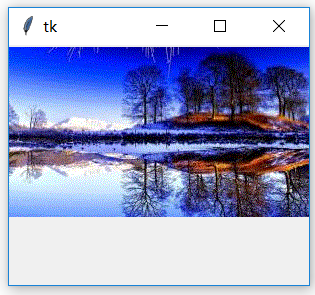4.1.3 图像与文字

``````import tkinter as tk

root=tk.Tk()
root.geometry('300x240')
p = tk.PhotoImage(file='a.gif')
b1 = tk.Label(root,image=p,text='图片说明',
compound='center',fg='yellow')
b1.pack()
root.mainloop()
``````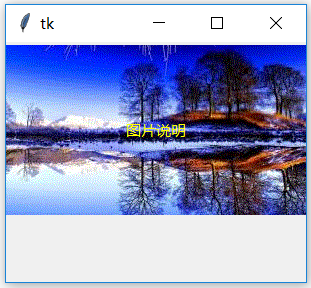4.1.4 多行文本

1. 手动插入换行符
``````import tkinter as tk
root=tk.Tk()
root.geometry('300x240')
b1 = tk.Label(root,
text='第一行\n第二行\n第三行')
b1.pack()
root.mainloop()
``````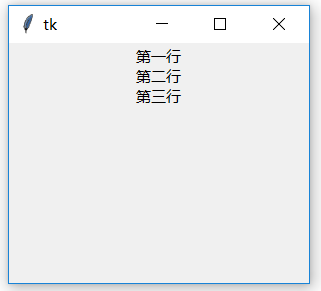2. wraplength

``````import tkinter as tk
root=tk.Tk()
root.geometry('300x240')
b1 = tk.Label(root,
text='这是一行很长的文字，\
我们可以使用wraplength来实现自动换行',
wraplength=200)
b1.pack()
root.mainloop()
``````